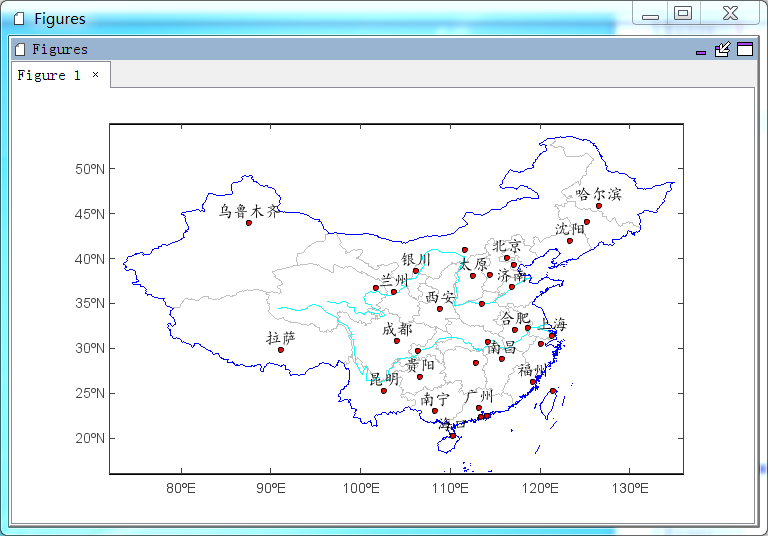Shape file is widely used GIS data format and there are many free online resouces. shaperead function is used to read shape file as a MILayer object with map coordinates and attribution data. The object can be added in an Axesm by geoshow function. A deault legend was defined when read shape file as a layer and it can be changed in geoshow function by setting facecolor, edgecolor, size and so on parameters. Also a more complex legen can be created using ‘makesymbolspec’ function. Following code will create a legend with two breaks of Yangtze and Huang He rivers.

ss = makesymbolspec('line', {'value':'Yangtze', 'color':(0,255,255), 'size':1}, \
{'value':'Huang He', 'color':(0,255,255), 'size':1}, field='NAME')
geoshow(river_layer, symbolspec=ss)


If a point layer was added, ‘labelfield’ and other associated parameters can be used to add labels to points.

#Plot
ax = axesm(tickfontsize=12)
geoshow('cn_province', edgecolor='lightgray')
geoshow('china', edgecolor=(0,0,255))
ss = makesymbolspec('line', {'value':'Yangtze', 'color':(0,255,255), 'size':1}, \
{'value':'Huang He', 'color':(0,255,255), 'size':1}, field='NAME')
geoshow('rivers', symbolspec=ss)
geoshow('cn_cities', facecolor='r', size=4, labelfield='NAME', fontname=u'楷体', \
fontsize=16, yoffset=15)
xlim(72, 136)
ylim(16, 55)